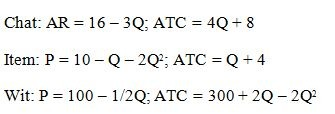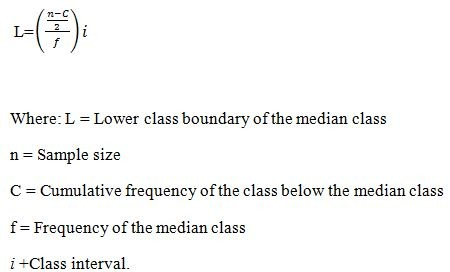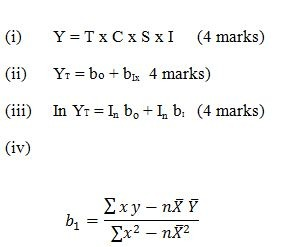# Quantitative Analysis Pilot Exam December 2021

CPA FOUNDATION LEVEL

CIFA FOUNDATION LEVEL

PILOT PAPER

QUANTITATIVE ANALYSIS

December 2021. Time Allowed: 3 hours.

Answer any FIVE questions. Marks allocated to each question are shown at the end of the question. Show ALL your workings.

QUESTION ONE

(a) Mashariki Enterprises started business in January 2018 selling photo copiers in the City. The following information relates to sales and enquiries made during the year ended 31 December 2020.
Sales calls (x)        Copiers sold (y)
96                                   41
40                                   41
104                                 51
128                                 60
164                                 61
76                                   29
72                                   39
88                                  50
36                                  28
84                                 43
180                               70
132                                56

Required:
Derive the regression equation of y on x. (12 marks)
(b) Explain four characteristics of Karl Pearson coefficient of correlation. (8 marks)
(Total: 20 marks)

QUESTION TWO

An electronics dealer in Nakuru has labelled a certain electrical component with numbers 1 – 50. These components are normally sold to 5 specific customers who pick one each on week days only. Incidentally, the components labelled numbers 3, 18, 12, 26 and 46 are defective.
Required:
(a) The probability that one customer will have drawn five defective components the end of 5 weeks. (15 marks)
(b) The probability that two customers will have drawn two defective components each, two none and the other components, in two weeks. (5 marks)
(Total: 20 marks)

QUESTION THREE
(a) Explain the difference between the following terms:
(i) Type 1 and Type II errors. (4 marks)
(ii) One-tail test and two-tail test. (4 marks)
(iii) Normal distribution and t-distribution. (4 marks)
(b) The manufacturer of the TyroX radical truck tyre claims that the mean mileage the tyre can be driven before the
thread wears out is 60,000 km, assuming the mileage wear follows the normal distribution and a standard deviation of 5,000 km. In a sample of 48 tyres, the mileage was found to be 59,500 km.
Required:
Test whether this observation is different from the claim the manufacturer at 5% significance level. (8 marks)
(Total: 20 marks)

QUESTION FOUR
Agro manufacturers produce three products; Chat, Item and Wit (in thousands) whose demand and cost functions are given as follows:Required:
(a) Output and price levels that will maximize profits. (9 marks)
(b) Maximum profit for each product. (9 marks)
(c) Total profit for the production of the three products at the optimal point. (2 marks)
(Total: 20 marks)

QUESTION FIVE
The frequency distribution of after tax earnings for Applewood Ltd. for 180 months to 31 December 2020 was as follows:

Profit after tax Sh“000” (X)           Frequency (f)
20,000≤ x < 60,000                                        8
60,000 ≤ x < 100,000                                    11
100,000≤ x < 140,000                                   23
140,000 ≤ x < 180,000                                  38
180,000 ≤ x < 220,000                                 45
220,000 ≤ x < 260,000                                 32
260,000 ≤ x < 300,000                                 19
300,000 ≤ x < 340,000                                  4
Required:
(a) Modify the formula given below for median to derive another one for:
(i) 25th percentile of the distribution. (6 marks)
(ii) 75th percentile of the distribution. (6 marks)
(Ensure to indicate what each of the symbols used stand for)
(b) Evaluate:
(i) 2nd decile of the distribution. (4 marks)
(ii) 8th decile of the distribution. (4 marks)(Total: 20 marks)

QUESTION SIX

(a) Write short notes on the following formulas in relation to time series analysis:(b) Explain the process of exponential smoothing. (4 marks)
(Total: 20 marks)

QUESTION SEVEN

In the context of linear programming, explain each of the following:
(a) Constrained optimisation. (4 marks)
(b) Inequality constraints. (4 marks)
(c) Objective function. (4 marks)
(d) Constrained minimisation. (4 marks)
(e) Non-negativity constraints. (4 marks)
(Total: 20 marks)